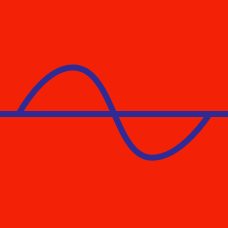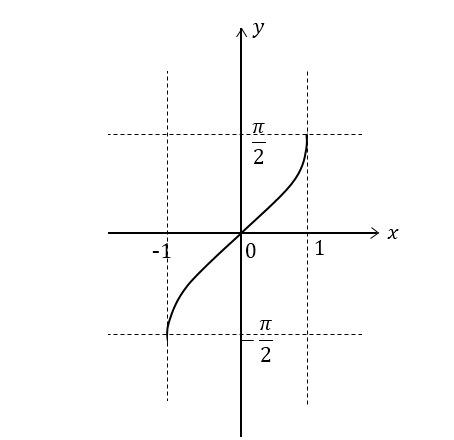Geometry

# Inverse Trigonometric Graphs

What is the $x$-coordinate of the intersection point between the graphs $y=\arccos x$ and $y=\frac{\pi}{2} ?$

What is the value of the $x$-coordinate of the intersection points between $y=\arcsin x$ and $y=\arccos x?$

What is the minimum value of the function $y= \lvert\arcsin x\rvert$ in the interval $-1 \leq x \leq 1?$

Let $y=\tan x$ for $-\frac{\pi}{2} < x < \frac{\pi}{2} .$ What is the range of the inverse function $\arctan x?$The above diagram shows the graph of which of the following inverse trigonometric functions?

×